## Example Questions

### Example Question #8 : Even / Odd Numbers

If m, n and p are odd integers, which of the following must be an odd integer?

m + n + p + 1

m * p * (n -1)

m * (n + p)

(m - 2 )* n * p

(m + 1) * n

(m - 2 )* n * p

Explanation:

When multiplying odd/even numbers, we know that odd * odd = odd, and odd * even = even. We also know that odd + odd = even. We will proceed to evaluate each answer choice, knowing that m, n and p are odd.

(m + 1) * n

m + 1 becomes even. This gives us even * odd = even.

m + n + p + 1

Odd + odd + odd + odd = even + odd + odd = even + even = even.

(m - 2 )* n * p

m - 2 is stil odd. This gives us odd * odd * odd = odd * odd = odd.

m * (n + p)

Odd + odd is even, so here we have odd * even = even.

m * p * (n -1)

n - 1 becomes even so we have odd * odd * even = odd * even = even.

The correct answer is therefore m * p * (n -1).

### Example Question #9 : Even / Odd Numbers

The operation ¤ is defined for all integers x and y as: x ¤ y = 4x-y2.

If x and y are positive integers, which of the following cannot produce an odd value?

x ¤ (y+1)

x ¤ y2

y ¤ x

x ¤ y

x ¤ 2y

x ¤ 2y

Explanation:

For this problem, we must recognize under what arithmetic conditions an even or odd number is produced. We do not know what the values for x and y are, but we do know for example that any two numbers added can be either even or odd, and and any number multiplied by two must be even (e.g. 2 * 2 = 4; 3 * 2 = 6), or an odd number multiplied by another odd is always even.

In this situation, we have to ensure that an arithmetic operation (subtraction) must result only in an even number, by further ensuring that the variables themselves are fixed to either odd or even values.

In this situation, we know that due to the "*2 (= 2 * 2)" principle, the 4x component always being an even value. The only situation in which the operation "4x – y2" will be even is if the "y2" term is also even, since an even minus an odd would be odd. The only situation in which the "y2" term is even is if the y itself is even, since an odd number squared always results in an odd value (e.g. 3= 9; 7= 25). To ensure that the y itself is even, we must also double it to "2y"(2).

We cannot add 1 to a random variable y as it may still result in an odd y-value. Similarly, since we already stated that a squared value may still be odd, we cannot be sure that squaring the y will also result in an even number.

### Example Question #10 : Even / Odd Numbers

What is the 65th odd number?

133

127

125

129

131

129

Explanation:

Doing this by counting the odd numbers will take way too long for the GRE.  And if you look at the answer choices, you see five consecutive odd numbers, so one little mistake in counting will give you the wrong answer!  Instead, we should use the formula for finding odd numbers: the nth odd number is 2n – 1.  (The nth even number is 2n.)  So the 65th odd number is 2 * 65 – 1 = 129.

### Example Question #11 : Even / Odd Numbers

Ifandare odd integers, andis even, which of the following must be an odd integer?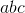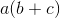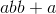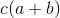Explanation:

Even numbers come in the form 2x, and odd numbers come in the form (2x + 1), where x is an integer.  If this is confusing for you, simply plug in numbers such as 1, 2, 3, and 4 to find that:

Any odd number + any even number = odd number

Any odd number + any odd number = even number

Any even number x any number = even number

Any odd number x any odd number = odd number

a(b + c) = odd x (odd + even) = odd x (odd) = odd

### Example Question #9 : How To Add Odd Numbers

At a certain high school, everyone must take either Latin or Greek. There aremore students taking Latin than there are students taking Greek. If there are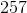students taking Greek, how many total students are there?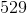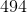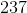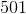Explanation:

If there arestudents taking Greek, then there are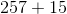or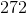students taking Latin. However, the question asks how many total students there are in the school, so you must add these two values together to get: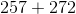ortotal students.

Tired of practice problems?

Try live online GRE prep today.